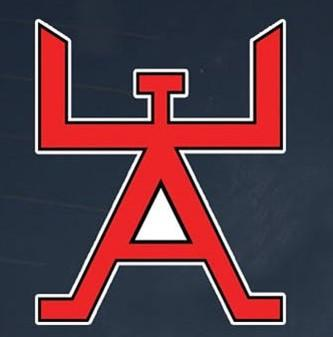# CDC 3e551 Engineering Journeyman

258 Questions | Total Attempts: 726SettingsAFSC 3E551 Edit Code 03

• 1.
Which reference ellipsoid surface do we use in the Air Force?
• A.

Geographic Coordinate System (GCS)

• B.

Universal Transverse Mercator (UTM)

• C.

• D.

World Geodetic System 1984 (WGS84)

• 2.
To represent the ellipsoid in two dimensions, we use a projection called
• A.

Geographic Coordinate System (GCS)

• B.

Universal Transverse Mercator (UTM)

• C.

• D.

World Geodetic System 1984 (WGS84)

• 3.
What do we call a projection that preserves angles, as opposed to shape, area, or distance?
• A.

Reference

• B.

Azimuthal

• C.

Conformal

• D.

Cylindrical

• 4.
A bearing of “South 60 degrees East” translates to an azimuth of
• A.

60

• B.

90

• C.

120

• D.

180

• 5.
What ratio is the most common stadia interval in the United States?
• A.

1:100

• B.

1:200

• C.

1:333

• D.

1:500

• 6.
What is parallel to lines of longitude from the Earth’s rotational axis?
• A.

Zenith

• B.

Bearing

• C.

Grid north

• D.

Magnetic north

• 7.
When correcting magnetic north to grid north, what do we do with an easterly declination?
• A.

Divide by longitude

• B.

• C.

Multiply by grid north

• D.

Subtract from magnetic north

• 8.
With a starting azimuth of 48 degrees after turning counterclockwise. What measurement is our new azimuth?
• A.

54

• B.

–54

• C.

160

• D.

306

• 9.
Because someone unfamiliar with surveying may use your field notes, the field notes must be
• A.

Clear and detailed

• B.

General and in English

• C.

Specific and in active voice

• D.

Locked in a box and protected from tampering

• 10.
Since field notes are legal documents and we do not erase anything, how do we mark corrections?
• A.

Line through error, note change above the line

• B.

Scratch out error, note change on separate page

• C.

Line through error, note change on separate page

• D.

Tear out page, start the survey over and begin a new page

• 11.
Where in our post-processing software can we find the feature definition manager?
• A.

Edit menu, under Tools, top of the list

• B.

File menu, under Tools, second on the list

• C.

File menu, under Options, last item on list

• D.

Survey menu, under Features, bottom of the list

• 12.
What six feature definition types are in the feature code libraries?
• A.

Point, line, polygon, group, array, and integer

• B.

Integer, decimal, character, string, array, and photo

• C.

Category, point, line, polygon, line control, and group

• D.

Category, point, line, polygon, polygon control, and group

• 13.
What feature code library command starts a line at a point?
• A.

Begin Line

• B.

Starts Line

• C.

First Point

• D.

Activate Line

• 14.
What do we call additional information we want to collect and assign to feature codes?
• A.

Features

• B.

Attributes

• C.

Descriptions

• D.

Characteristics

• 15.
What are the four file types for feature code libraries?
• A.

Shapefile, database, .job file, and Nikon code list

• B.

Simple text, comma delimited, image, and CAD drawing

• C.

HTML file, XML file, data dictionary file, and simple text

• D.

Simple text, Nikon code list, data dictionary file, and feature/attribute library

• 16.
At what decimal feet must the 2-peg test results be before the instrument needs calibration?
• A.

.08

• B.

.18

• C.

.25

• D.

.125

• 17.
Where in the controller menus can you find the horizontal angle (HA)/vertical angle (VA) collimation test function?
• A.

• B.

Main menu, then Survey, then Measure points

• C.

• D.

Main menu, then Instrument, then Tests

• 18.
What equation is used to convert millimeters (mm) to feet (ft.)?
• A.

mm / 3.2808 = ft

• B.

Mm * 3.2808 = ft

• C.

Mm / 0.0032808 = ft.

• D.

Mm * 0.0032808 = ft

• 19.
Which US Army Corps of Engineers (USACE) engineering manual (EM) has comprehensive instructions on monument installation?
• A.

10–401

• B.

100–5–0001

• C.

1110–1–1005

• D.

1110–A–1005

• 20.
How many years are temporary monument markers expected to last?
• A.

One

• B.

Two

• C.

Three

• D.

Four

• 21.
Regarding installing survey monuments, what are the two most common soil types?
• A.

A and D

• B.

B and G

• C.

C and G

• D.

D and E

• 22.
For which materials are bronze disk monuments best suited?
• A.

Steel and wood

• B.

Sands and gravels

• C.

Concrete and asphalt

• D.

Bedrock or concrete permanent structures

• 23.
Convert 30 10’20” into decimal degrees to three decimals.
• A.

30.17

• B.

30.15

• C.

29.17

• D.

29.11

• 24.
When adjusting a traverse, which columns do we total to get the misclosure?
• A.

Angle and distance

• B.

Latitude and departure

• C.

Latitude and longitude

• D.

Northings and eastings

• 25.
How do we decide the proportion of misclosure a latitude or departure receives?
• A.

• B.

Station number

• C.

Portion of interior angles

• D.

Proportion of horizontal distance

Related TopicsBack to top